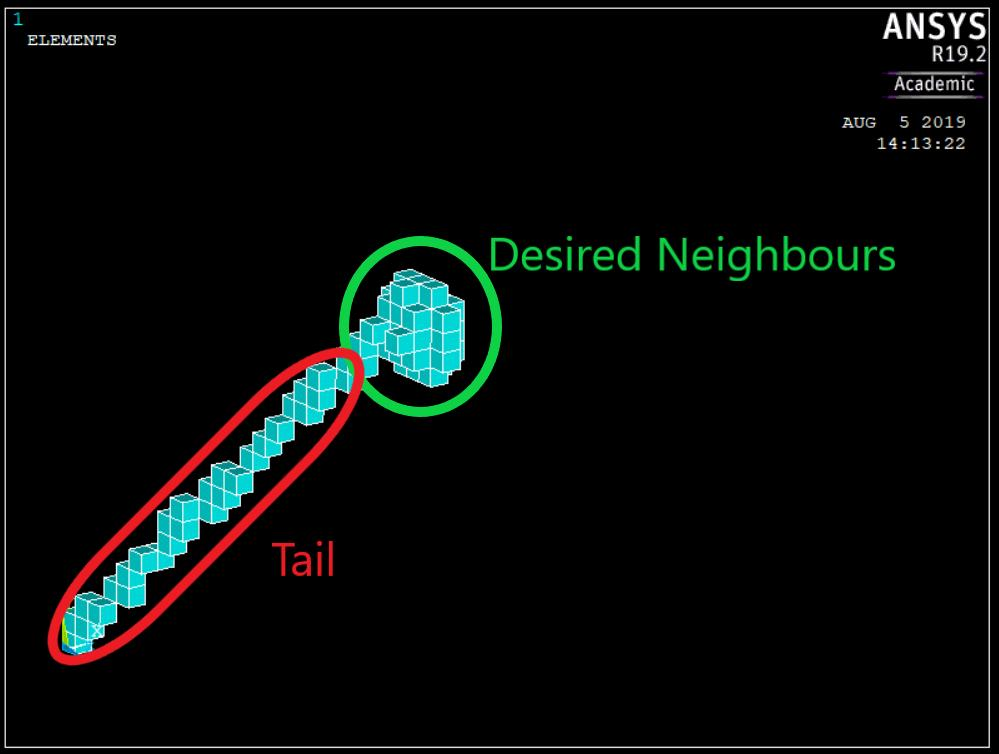# PPATH function includes the origin in every path defined

Hi all,

I have meshed a general block volume and I'm trying to use PATH along with ESEL to select elements in the neightbourhood (of radius "R") of a given element "i".

I use *DO to insert the X Y Z coords of a spherical path around " i " into PPATH,

then I issue ESEL,S,path,"MYPATH" to select elements, the expected result is a set of elements containing those around "i".

the problem is that, although the array of coordinates XYZ does not contain the value (0,0,0), the selected set of elements includes a "tail" of elements that goes from the origin to the element "i" as if the path defined by PPATH wasn't only a sphere of points but the sphere plus the origin, and that behavior holds for any element.

Code used:

/PREP7

*AFUN, DEG

!Dimensões do bloco

L = 0.1 ! Lado [m]

x1 = 0

y1 = 0

z1 = 0

x2 = L

y2 = L

z2 = L

BLOCK, X1, X2, Y1, Y2, Z1, Z2

E _0= 2E11

Poiss = 0.3

ET, 1,SOLID185

MP, EX, 1, E_0

MP, PRXY, 1,Poiss

save

n_div = 30

ESIZE,,n_div

VMESH, 1,

i = 15879 ! "i-th" element

loc_ei_x = CENTRX(i)

loc_ei_y = CENTRY(i)

loc_ei_z = CENTRZ(i)

PATH, Viz, 70,30, 50

R_viz = (L/n_div)*(3**(1/2)) !Setting Neightbourhood radius

!Filling array of spherical coords phi and theta

ang_phi(1:21) = 0

*DO, i, 2,21,

ang_phi(i) = ang_phi(i-1) + 18

*ENDDO

ang_teta(1) = 0,120,240

!Creating arrays to store values of the x y z coords inserted into path for verification
*DIM,x_path, ARRAY, 21, 3

*DIM,y_path, ARRAY, 21, 3

*DIM,z_path, ARRAY, 21, 3

!Inserting x y z into PPATH

k = 1

*DO, i, 1,21,

*DO, j, 1,3,

x_path(i,j) =  loc_ei_x + R_viz*cos(ang_phi(i))*sin(ang_teta(j))

y_path(i,j) = loc_ei_y  + R_viz*sin(ang_phi(i))

z_path(i,j) = loc_ei_z  + R_viz*cos(ang_phi(i))*cos(ang_teta(j))

PPATH, k, , x_path(i,j), y_path(i,j), z_path(i,j)

k = k+1

*ENDDO

*ENDDO

ESEL, S, path, Viz,

Result: